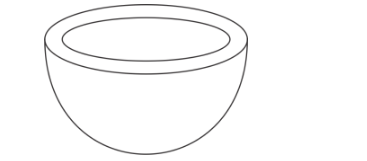# The internal and external diameters of a hollowQuestion:

The internal and external diameters of a hollow hemispherical vessel are 21 cm and 25.2 cm respectively. The cost of painting 1 cm2 of the surface is 10 paise. Find the total cost to paint the vessel all over.

Solution:

We are given the following hemi hollow sphereThe internal and external radii of the hollow hemispherical vessel are $\frac{21}{2}=10.5 \mathrm{~cm}$ and $\frac{25.2}{2}=12.6 \mathrm{~cm}$ respectively. Therefore, the total surface area of the hollow hemispherical vessel is

$S=2 \pi \times(12.6)^{2}+2 \pi \times(10.5)^{2}+\pi\left\{(12.6)^{2}-(10.5)^{2}\right\}$

$=2 \times \frac{22}{7} \times 158.76+2 \times \frac{22}{7} \times 110.25+\frac{22}{7} \times 48.51$

$=997.92+693+152.46$

$=1843.38$

The cost of painting 1 square cm is 10 paise. Therefore the total cost of painting the vessel all over is

$1843.38 \times 10=1843.38 \times 10$ Paise

$=184.338$ Rupees

Hence total cost of painting is $184.338$ rupees